# Atomic Structure and Periodic TrendsPage 8

#### WATCH ALL SLIDES

Make multi electron atom look like a one electron atom

Assumes every electron to be on its own experiencing an effective nuclear charge, Zeff

Then the orbitals for the electrons take the form of those in hydrogen but their energies and sizes are modified by simply using an effective nuclear charge

One electron atom

2+

-

-

-

-

Zeff

Two-electron atom

Orbital Approximation

Slide 66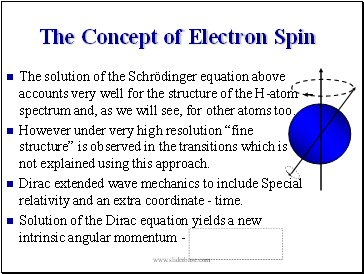The Concept of Electron Spin

The solution of the Schrödinger equation above accounts very well for the structure of the H-atom spectrum and, as we will see, for other atoms too.

However under very high resolution “fine structure” is observed in the transitions which is not explained using this approach.

Dirac extended wave mechanics to include Special relativity and an extra coordinate - time.

Solution of the Dirac equation yields a new intrinsic angular momentum -

Slide 67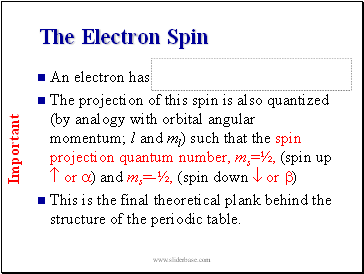The Electron Spin

An electron has

The projection of this spin is also quantized (by analogy with orbital angular momentum; l and ml) such that the spin projection quantum number, ms=½, (spin up  or a) and ms=-½, (spin down  or b)

This is the final theoretical plank behind the structure of the periodic table.

Important

Slide 68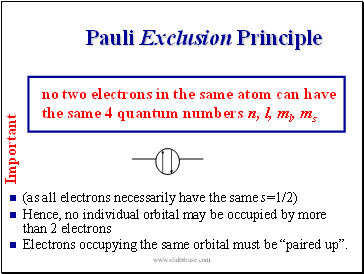Pauli Exclusion Principle

(as all electrons necessarily have the same s=1/2)

Hence, no individual orbital may be occupied by more than 2 electrons

Electrons occupying the same orbital must be “paired up”.

Important

no two electrons in the same atom can have the same 4 quantum numbers n, l, ml, ms

Slide 69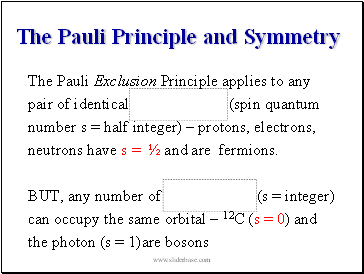The Pauli Principle and Symmetry

The Pauli Exclusion Principle applies to any

pair of identical (spin quantum

number s = half integer) – protons, electrons,

neutrons have s = ½ and are fermions.

BUT, any number of (s = integer)

can occupy the same orbital - 12C (s = 0) and

the photon (s = 1)are bosons

Slide 70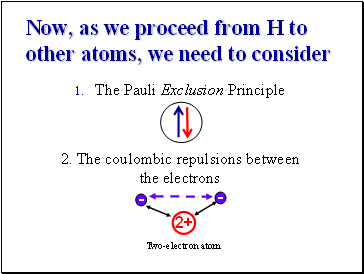Now, as we proceed from H to other atoms, we need to consider

The Pauli Exclusion Principle

2. The coulombic repulsions between the electrons

2+

-

-

Two-electron atom

Slide 71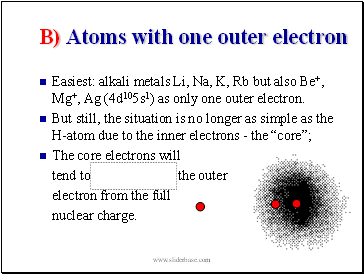B) Atoms with one outer electron

Easiest: alkali metals Li, Na, K, Rb but also Be+, Mg+, Ag (4d105s1) as only one outer electron.

But still, the situation is no longer as simple as the H-atom due to the inner electrons - the “core”;

Go to page:
1  2  3  4  5  6  7  8  9  10  11  12  13  14  15
16  17